The goal for this lab is to introduce you to Ordinary Least Squares regression applications in R.

# 1. Fitting Linear Models

Ordinary Least Squares (OLS) regression, also known as simple/linear regression, is the workhorse model for statistical hypothesis testing in the social sciences. While not as widely used as it once was, linear models often serve as a useful reference point for ones study that may go on to use more complex linear and nonlinear modeling techniques. Additionally, linear models are useful in a variety of physical science and machine learning applications.

Linear regression, like any statistical model, is merely a collection of mathematical and philosophical assumptions that allow us to restrict our data to a simple functional form. Assuming that your process of interest is appropriately modeled by restricted functional form assumptions carries both advantages and disadvantages. From an advantage standpoint, using a linear model helps to improve interpretation through the simplicity of the model assumptions. Conversely, using a linear functional form could hold many disadvantages for modeling real-world data. If our data does not realistically fit into our assumptions behind the linear process, than it is likely that our findings will be biased to the extent by which the real data generation process deviates from a linear form. I will not go over all of the mechanics of the linear model, but I will quickly articulate the main set of assumptions that underlay the linear model below.

Appropriate variable specification
your linear model should include the correct covariates and should not have omitted variables.
Strict exogeneity
$$E[\epsilon|X]=0$$
the model errors should have a conditional mean of zero.
your right hand side covariates should be uncorrelated with the error term.
if exogeneity doesn’t hold, then your model variables are said to be endogenous.
No linear dependence among right hand covariates.
your independent variables should not be perfectly collinear.
also known as multicollinearity.
if your regressors are perfectly collinear, then it is impossible to truly know $$\beta$$.
Spherical errors
$$Var[\epsilon | X] = \sigma^{2}I_{n}$$
$$\sigma^{2}$$ is treated as a nuisance parameter that measures the variance for each observation.
errors are spherical if two conditions occur in the error term, homoscedasticity and no autocorrelation.
homoscedasticity ($$E[\epsilon_{i}^{2}|X] = \sigma^{2}$$) indicates that each observation has the same variance ($$\sigma^{2}$$) for each observation.
No Autocorrelation ($$E[\epsilon_{i}\epsilon_{j}|X] = 0$$ or $$i \not= j$$), which specifies that errors are uncorrelated across observations.

The above assumptions are also often specified as the Independent and Identically distributed (iid) assumption behind linear models. Notice that almost every assumption deals with issues of the linear model’s error term. This is because the linear model seeks to minimize the residual error left over after taking into account your model covariates. If you leave important covariates out, specify covariates incorrectly, or fail to adequately take into account the data generation process, then your model will be unable to adequately minimize the leftover error from your model specification. In addition to these assumptions, it is often useful to think about the probability distribution of your data. If your linear estimator has normally distributed errors, it is equivalent to maximum likelihood. In addition, you should think about the theoretical connection between your covariates and the dependent variable. Remember that you are assuming a linear functional relationship between your right hand variables ($$x'$$) and the dependent variable ($$y$$).

## Simple Linear Models in R

Now that we have reoriented ourselves with linear models, lets fit some simple linear regressions. Remember that a simple linear model is merely one dependent variable ($$y$$) and one independent variable ($$x$$). Let’s first load some useful library packages for this exercise.1

library(MASS)
library(ISLR)
library(car)
library(effects)
##
## Attaching package: 'effects'
##
## The following object is masked from 'package:car':
##
##     Prestige

The car package has a useful data set called Davis, which measures the heights and weights of 200 men and women who are engaged in exercise. Lets explore this data set a bit further.

fix(Davis)

names(Davis)

?Davis

All of the above commands serve as useful ways to examine data frames. The fix() function opens up an edit window for the data set, and then loads the data set into the global environment after you close the window. The other three commands should be more familiar to you, but you are probably wondering about the ?Davis statement. Using the ? in front of an object name will pull up a helpful description of the objects purpose and background. Quickly read through the description for the Davis data set.

The weight variable is a participants recorded weight, and the repwt variable is a participants self-reported weight. Let’s say we want to see how predictive self-reported weight is of actual weight. To do this, we can start with the general equation below:

$E(y|x_{1},...,x_{k}) = \beta_{0} + \beta_{1}x_{1} + ... + \beta_{k}x_{k} + \epsilon$

If you are not strong in statistics, the above equation can be a bit daunting, but it is actually quite simple! $$E(y|x_{1},...,x_{k})$$ simply refers to the expected value ($$E$$) of your dependent variable ($$y$$) given all of your right hand side covariates ($$x_{1},...,x_{k}$$, with k being total number of variables specified). $$\beta_{0}$$ is the intercept parameter, which is also called the constant. The constant simply specifies the mean value of $$y$$ given the right hand side covariates. $$\beta_{1}$$ through $$\beta_{k}$$ is simply a list of estimated slope coefficients for all included covariates ($$x_{k}$$). Finally, $$\epsilon$$ corresponds to the, hopefully, well-behaved error term that represents an iid process. If we want to estimate a simple linear model that uses self-reported weight (repwt) as a predictor of actual weight (weight), than we can use a simplified version of this general equation:

$E(weight|repwt) = \beta_{0} + \beta_{1}repwt + \epsilon$

This equation states that the expected value of weight is dependent on a linear combination of mean weight ($$\beta_{0}$$) and reported weight ($$\beta_{1}repwt$$). Lets go ahead and extimate this modeling using R.

model <- lm(weight ~ repwt, data=Davis)

model 
##
## Call:
## lm(formula = weight ~ repwt, data = Davis)
##
## Coefficients:
## (Intercept)        repwt
##      5.3363       0.9278
summary(model)
##
## Call:
## lm(formula = weight ~ repwt, data = Davis)
##
## Residuals:
##     Min      1Q  Median      3Q     Max
##  -7.048  -1.868  -0.728   0.601 108.705
##
## Coefficients:
##             Estimate Std. Error t value Pr(>|t|)
## (Intercept)   5.3363     3.0369   1.757   0.0806 .
## repwt         0.9278     0.0453  20.484   <2e-16 ***
## ---
## Signif. codes:  0 '***' 0.001 '**' 0.01 '*' 0.05 '.' 0.1 ' ' 1
##
## Residual standard error: 8.419 on 181 degrees of freedom
##   (17 observations deleted due to missingness)
## Multiple R-squared:  0.6986, Adjusted R-squared:  0.697
## F-statistic: 419.6 on 1 and 181 DF,  p-value: < 2.2e-16

R uses the lm() function to estimate linear models. In this example we housed the function results in an object called model. If you print the model object, you get a sparse description of the formula and model coefficients. If you use the summary() function on the model object, you get a more complete analysis of the residuals, coefficients, and model fit statistics. Notice that data concerning the confidence intervals are missing, but that can be obtained using the confint() function.

confint(model)
##                  2.5 %    97.5 %
## (Intercept) -0.6560394 11.328560
## repwt        0.8384665  1.017219

Examining both the main regression results and the confidence intervals raises a couple of red flags. First, if reported weight is predictive of actual weight, then the coefficient for $$\beta_{0}$$ should be closer to 0 and the coefficient for $$\beta_{1}$$ should be closer to 1. The slope estimate for reported weight seems to reach towards this ideal, but the intercept is well off from the expected. The residual standard error is also relatively large, while the adjusted $$R^{2}$$ seems low for measures that should be highly correlated. What could be going on?

It is also useful to graph the results of the regression using the scatterplot() function. It is possible that there is an outlier that is influencing the results away from reality, and this will be easy to examine from a visual perspective.

scatterplot(weight ~ repwt, data=Davis, smooth=FALSE, id.n=1)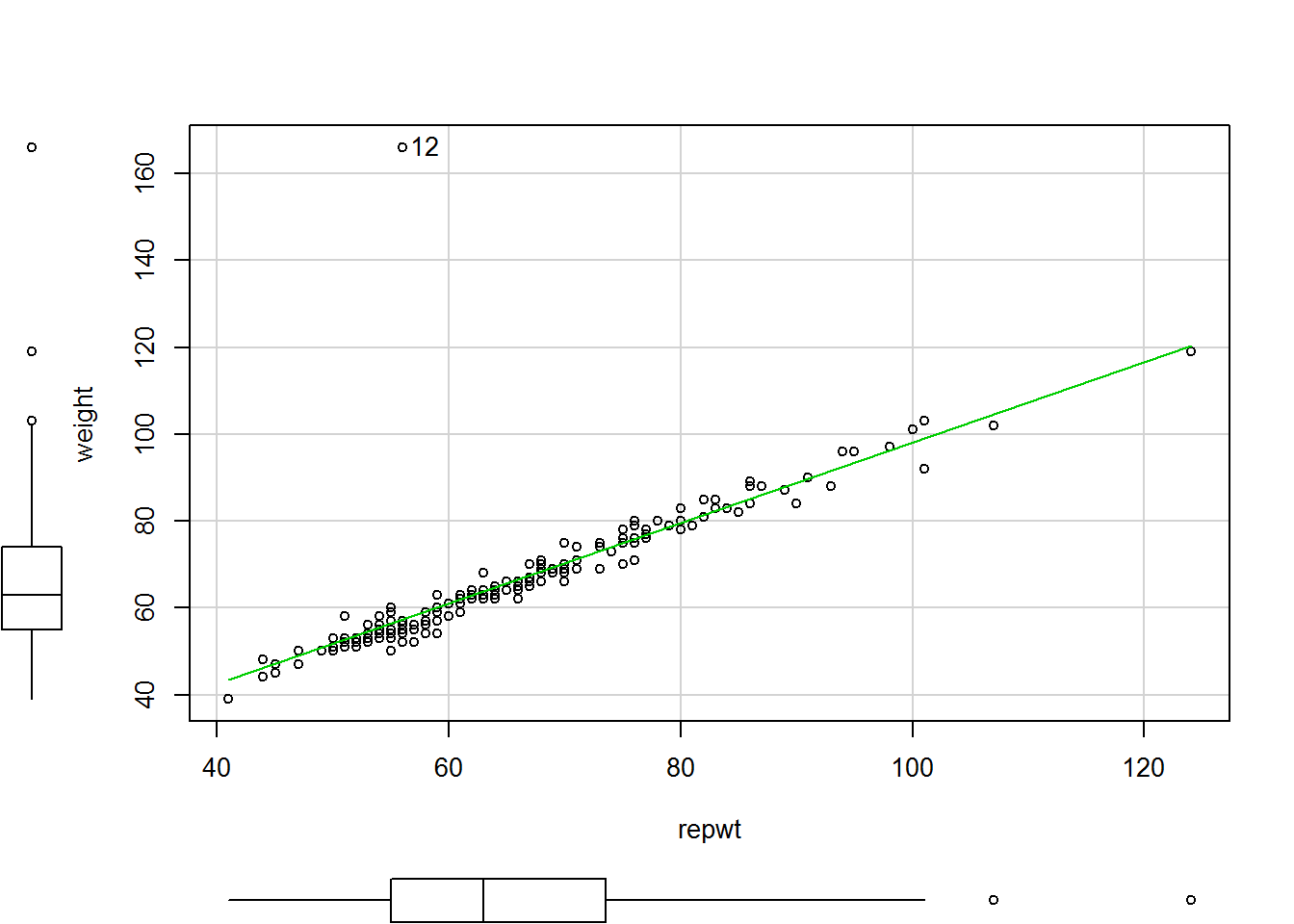## 12
## 12

Just as expected! Observation 12 has a significantly higher actual weight measure (~160kg) than its reported weight measure (~60kg). The id.n argument in the scatterplot code told the function to label one observation, with the default being to label extreme (outlier) observations first. Let’s re-estimate the model without the outlier observation.

model2 <- update(model, subset=-12)

summary(model2)
##
## Call:
## lm(formula = weight ~ repwt, data = Davis, subset = -12)
##
## Residuals:
##     Min      1Q  Median      3Q     Max
## -7.5296 -1.1010 -0.1322  1.1287  6.3891
##
## Coefficients:
##             Estimate Std. Error t value Pr(>|t|)
## (Intercept)  2.73380    0.81479   3.355 0.000967 ***
## repwt        0.95837    0.01214  78.926  < 2e-16 ***
## ---
## Signif. codes:  0 '***' 0.001 '**' 0.01 '*' 0.05 '.' 0.1 ' ' 1
##
## Residual standard error: 2.254 on 180 degrees of freedom
##   (17 observations deleted due to missingness)
## Multiple R-squared:  0.9719, Adjusted R-squared:  0.9718
## F-statistic:  6229 on 1 and 180 DF,  p-value: < 2.2e-16

The update() function is useful as it help you to avoid rewriting long model code over and over again. The code above simply took our previous model and deleted the 12th observation from the data. Eyeballing the new regression estimation summary shows that the results are much more in line with our empirical expectations. The residual standard error decreased from 8kg to 2.2kg. The adjusted $$R^{2}$$ has increased from .69 to .97, and the intercept coefficinet has decreased greatly.

## Multiple Linear Regression

When performing multiple linear regression, the formula remains analagous to the simple linear model specified above. Let’s revist the job prestige data using prestige as a dependent variable, and education, income, and women as right hand covariates.

$E(y|x_{1},x_{2},x_{3}) = \beta_{0} + \beta_{1}education + \beta_{2}log2(income) + \beta_{3}women + \epsilon$

prestige.model <- lm(prestige ~ education + log2(income) + women,
data = Prestige)

summary(prestige.model)
##
## Call:
## lm(formula = prestige ~ education + log2(income) + women, data = Prestige)
##
## Residuals:
##     Min      1Q  Median      3Q     Max
## -17.364  -4.429  -0.101   4.316  19.179
##
## Coefficients:
##               Estimate Std. Error t value Pr(>|t|)
## (Intercept)  -110.9658    14.8429  -7.476 3.27e-11 ***
## education       3.7305     0.3544  10.527  < 2e-16 ***
## log2(income)    9.3147     1.3265   7.022 2.90e-10 ***
## women           0.0469     0.0299   1.568     0.12
## ---
## Signif. codes:  0 '***' 0.001 '**' 0.01 '*' 0.05 '.' 0.1 ' ' 1
##
## Residual standard error: 7.093 on 98 degrees of freedom
## Multiple R-squared:  0.8351, Adjusted R-squared:   0.83
## F-statistic: 165.4 on 3 and 98 DF,  p-value: < 2.2e-16
confint(prestige.model)
##                      2.5 %      97.5 %
## (Intercept)  -140.42113183 -81.5105164
## education       3.02724622   4.4337694
## log2(income)    6.68224035  11.9470925
## women          -0.01243818   0.1062285
coef(prestige.model)
##   (Intercept)     education  log2(income)         women
## -110.96582409    3.73050783    9.31466643    0.04689514
cbind(Estimate=coef(prestige.model), confint(prestige.model))
##                   Estimate         2.5 %      97.5 %
## (Intercept)  -110.96582409 -140.42113183 -81.5105164
## education       3.73050783    3.02724622   4.4337694
## log2(income)    9.31466643    6.68224035  11.9470925
## women           0.04689514   -0.01243818   0.1062285

The coef() function allows you to extract the coefficient estimates from the linear model, just as the confint() does for confidence intervals. The cbind() function above simply combines the coefficient estimates with the confidence intervals into a simple table.

Using the effects package and the allEffects() function, we can examine the effect of our covariates with all other factors held at their mean levels.

plot(allEffects(prestige.model, default.levels=50), ask=FALSE)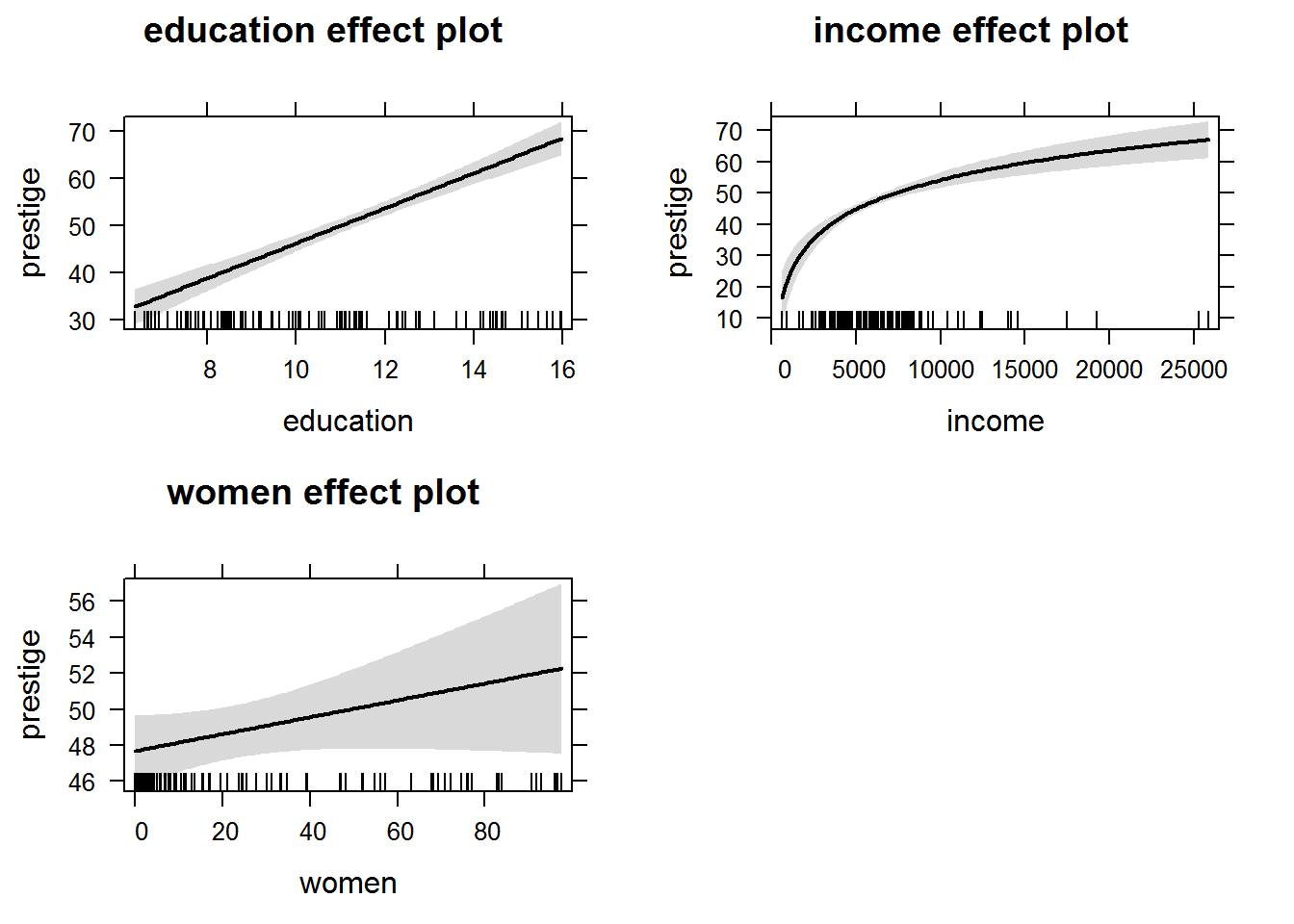The results show that education and income have strong increasing effects on prestige, but that the number of women does not. Take a look back to the regression summary. The grey confidence bands within the individual effect plots corresponds to the expected significance level of the covariate.

Finally, one can quickly examine residual diagnostics by simply specifying the plot() function on the regression model object. We will go over residuals in more depth later in the workshop, but this is a good starting point for thinking about diagnostics in R.

plot(prestige.model)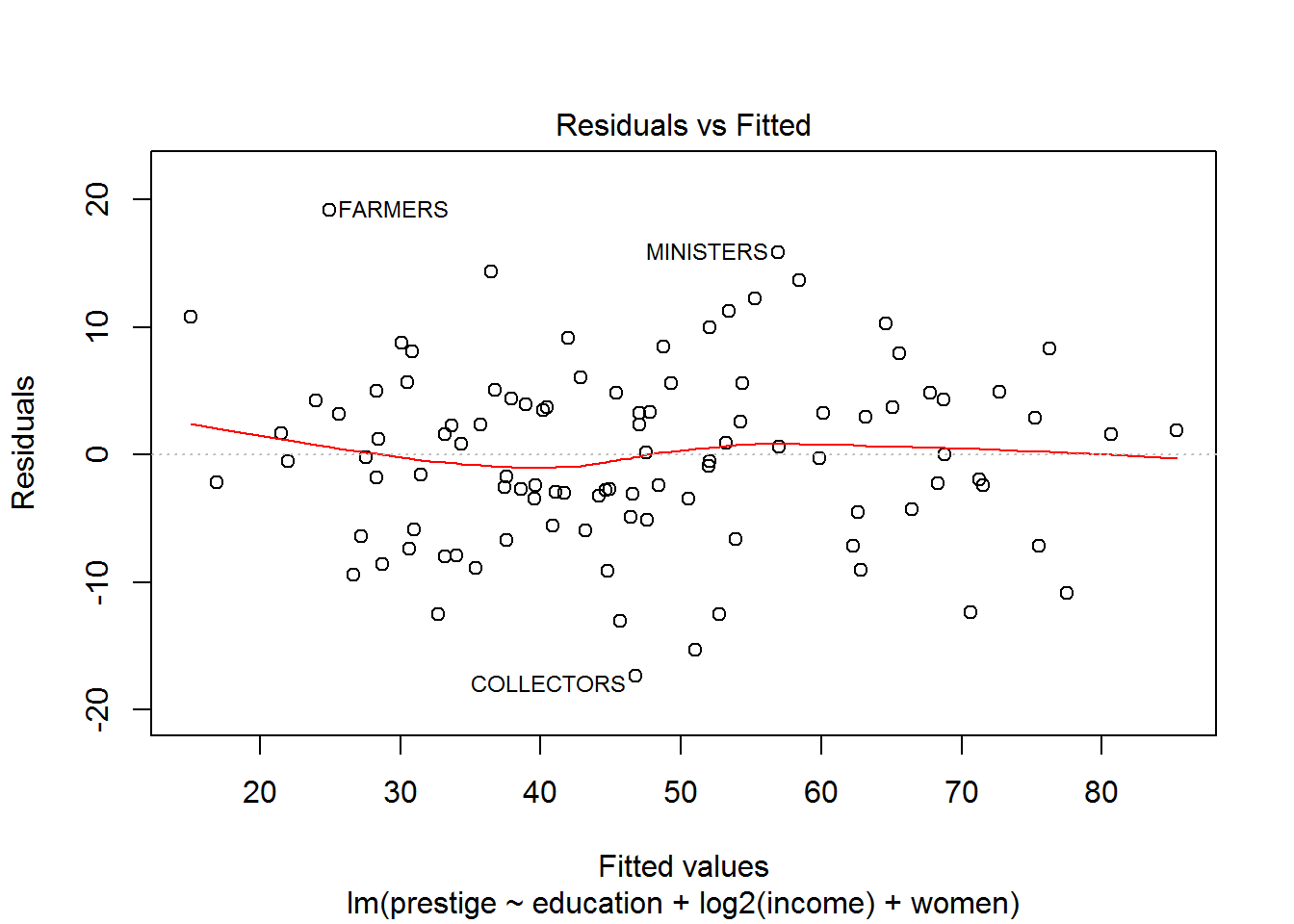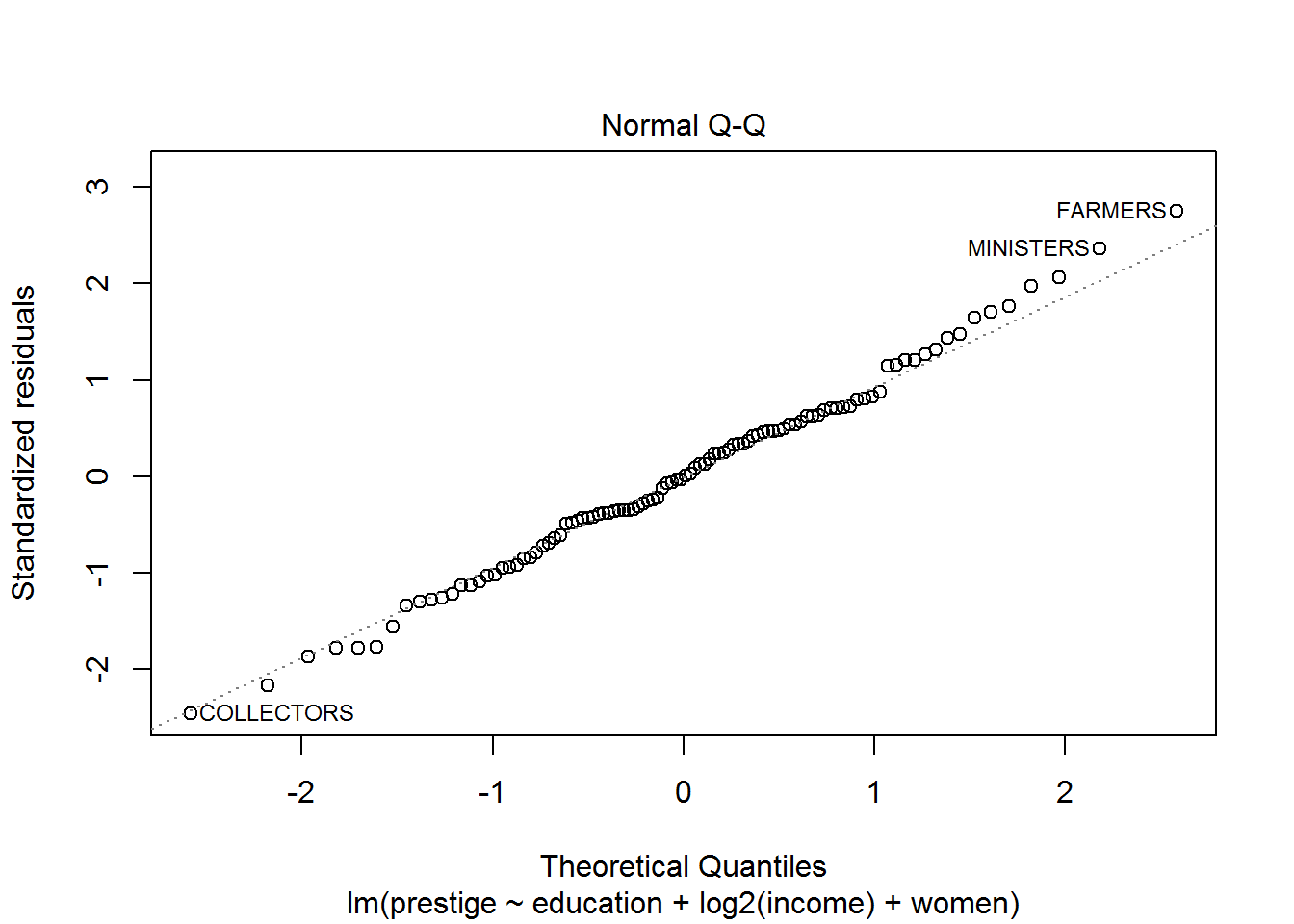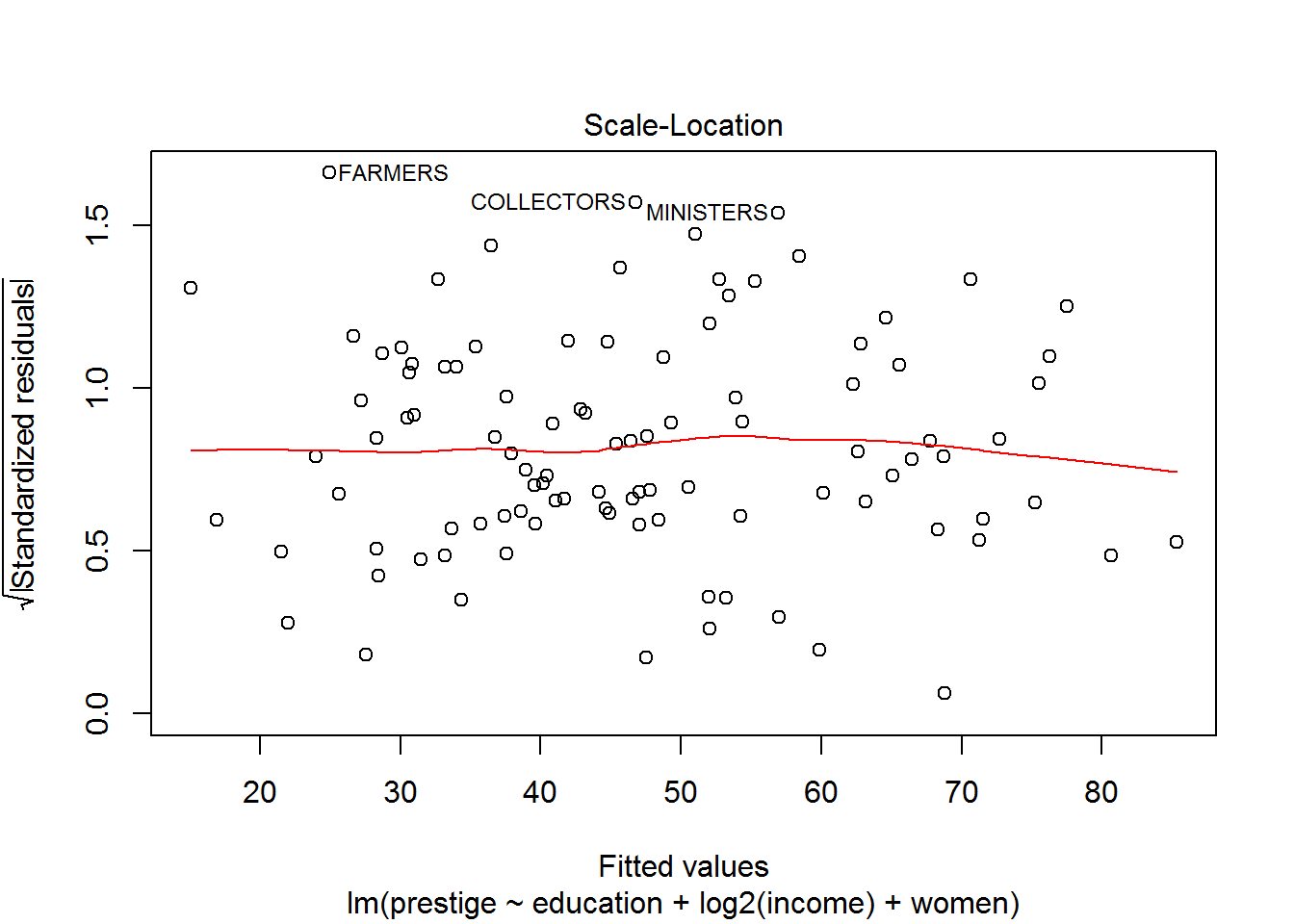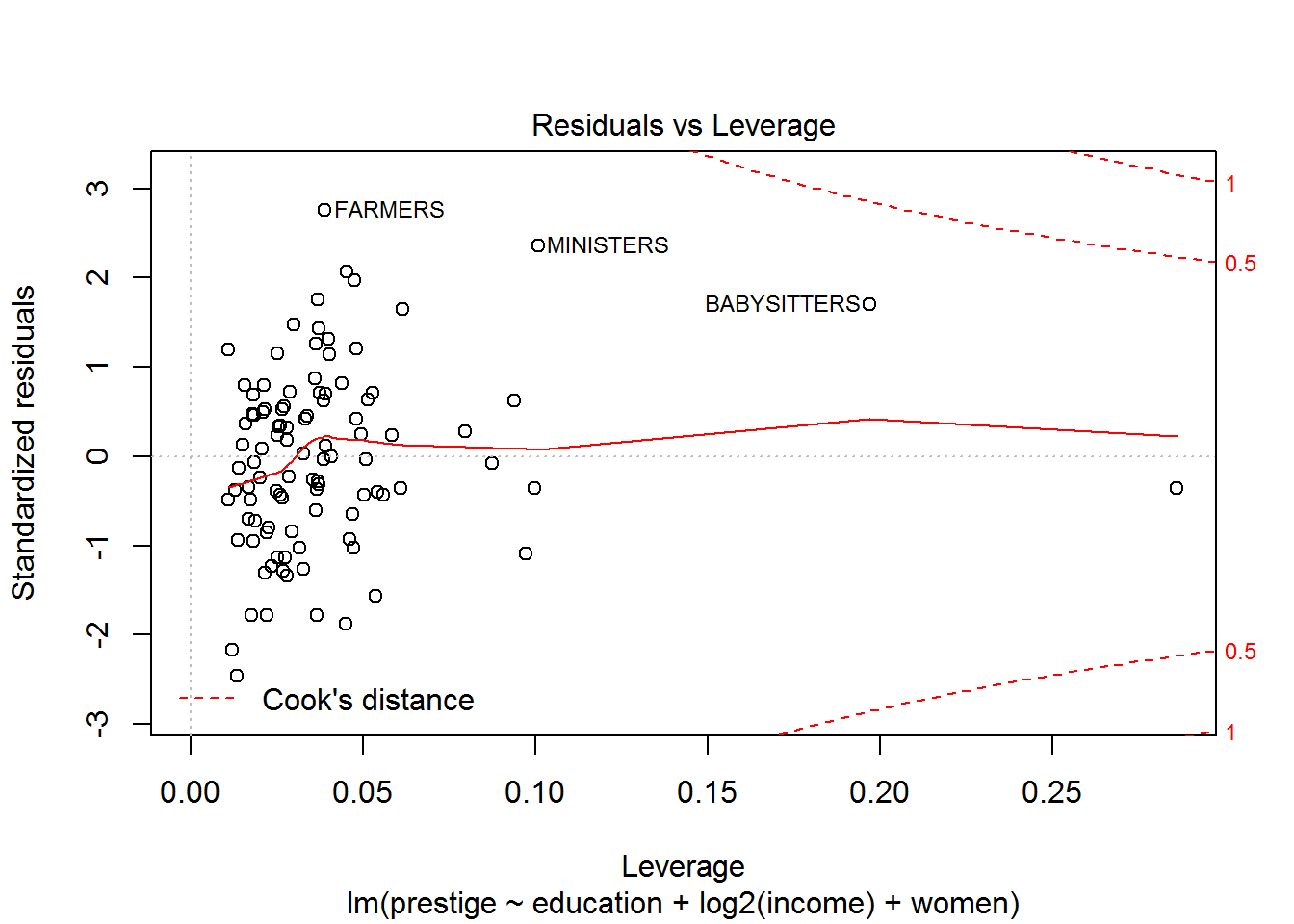1. Go ahead and download MASS, ISLR, effects, and car packages if you haven’t already.